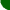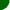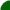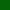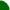welcome/ java-mcmc/ software/ papers/ links/ email mesitemap software essays mathematics unix links

Below are some research articles I have written or co-authored. The focus is on Markov Processes and their applications to large scale scientific computation problems. To illustrate some of these ideas, I have also written several Markov Chain demonstration applets in Java.

Web graph compression in MarkovPR 1.1 (2002)
abstract: These notes describe the results of some investigations into reducing the memory requirements of the MarkovPR 1.0 software for storing, and simulating from, massive web graphs. The statistics which illustrate our results are based upon the First Google Programming Contest dataset. Many of the techniques described here have been implemented in the new version, MarkovPR 1.1.
source code (licensed under GPL): markovpr-1.1.tar.bz2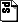ripcompress.ps.gz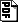ripcompress.pdf

Markovian page ranking distributions: some theory and simulations (2002)
abstract: We discuss some simple theoretical properties of web page ranking distributions, including PageRank (Brin and Page, 1998), which are based on uniformly ergodic Markov chains. We also propose a modification of PageRank with reduces the bias for older documents, and discuss details of our simulation programs.
source code (licensed under GPL): markovpr-1.01.tar.gzgooglerip.ps.gzgooglerip.pdf

Some directions in Perfect Simulation (2000)
abstract: Presentation given at the First European Conference on Spatial Statistics, Ambleside (17-22 september, 2000). The slides here have been slightly modified for web viewing. You can find the LaTeX source code and instructions for creating the slides here.ambleside2.pdf

A note on geometric ergodicity and floating-point roundoff error (2000)
coauthored with Gareth Roberts and Jeff Rosenthal
abstract: We consider the extent to which Markov chain convergence properties are affected by the presence of computer floating-point roundoff error. Both geometric ergodicity and polynomial ergodicity are considered. This paper extends previous work of Roberts, Rosenthal and Schwartz (1998); connections between that work and the present paper are discussed.glj8.ps.gzglj8.pdf

Catalytic perfect simulation (2000)
coauthored with Gareth Roberts
abstract: We introduce a methodology for perfect simulation using so called catalysts to modify random fields. Our methodology builds on a number of ideas previously introduced by Breyer and Roberts (1999), by Murdoch (1999), and by Wilson (1999). We illustrate our techniques by simulating two examples of Bayesian posterior distributions.simulations5.ps.gzsimulations5.pdf

slides also available:catalytic_slides.ps.gzcatalytic_slides.pdf

Some multi-step coupling constructions for Markov chains (2000)
coauthored with Gareth Roberts
abstract: We describe some old and new methods for coupling the flow of a discrete time Markov chain, provided its transition function is known. Examples including Hastings-Metropolis and Gibbs samplers are given.multistep.ps.gzmultistep.pdf

On the approximation of certain probability measures by a set of points (2000)
abstract: In this paper we describe a framework for the comparison of a finite set of points with a probability measure if the latter belongs to a simple class. The measure of closeness chosen quantifies the degree of agreement obtained when a prescribed collection of test functions is simultaneously integrated with respect to the given probability measure, and the set of points (identified with a sum of point masses). No specific assumptions are made about the provenance of the point set, although our results have a clear application to certain Markov chain Monte Carlo integration problems.hypo.ps.gzhypo.pdf

Convergence of simulated annealing using Foster-Lyapunov criteria (2000)
coauthored with Christophe Andrieu and Arnaud Doucet
abstract: Simulated annealing is a popular and much studied method for maximizing functions on finite or compact spaces. For noncompact state spaces, the method is still sound, but convergence results are scarce. We show here how to prove convergence in such cases, for Markov chains satisfying suitable drift and minorization conditions.ANNEAL.ps.gzANNEAL.pdf

Efficient Monte Carlo for neural networks with Langevin samplers (1999)
coauthored with Mauro Piccioni and Sergio Scarlatti
abstract: We consider the task of simulating efficiently from the posterior distribution over weight space of a feed-forward neural network using a Langevin-Metropolis sampler, given a finite data set. It is shown that as the number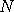of hidden neurons increase, the proposal variance must scale as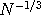in order to get convergence of the underlying discretized diffusions. This generalizes previous results of Roberts and Rosenthal (1998) for the i.i.d. case, shows robustness of their analysis, and also has practical applications.neural14.ps.gzneural14.pdf

A new method for coupling random fields (1999)
coauthored with Gareth Roberts
abstract: Given a Markov chain, a stochastic flow which simultaneously constructs sample paths started at each possible initial value can be constructed as a composition of random fields. In this paper, we describe a method for coupling flows by modifying an arbitrary field (consistent with the Markov chain of interest) by an independence Metropolis Hastings iteration. We show that the resulting stochastic flow has many desirable coalescence properties, regardless of the form of the original flow.fcoupler.ps.gzfcoupler.pdf

slides also available:poster99-2.ps.gzposter99-2.pdf

Quasistationarity and the relative entropy of probability measures (1999)
abstract: Consider a Markov process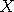with finite lifetime. In this paper, we derive sufficient conditions for the convergence of the law of, conditioned on longer and longer lifetimes, using information theoretic techniques.quasism.ps.gzquasism.pdf

From Metropolis to diffusions: Gibbs states and optimal scaling (1998)
coauthored with Gareth Roberts
abstract: This paper investigates the behaviour of the random walk Metropolis algorithm in high dimensional problems. Here we concentrate on the case where the components in the target density is a spatially homogeneous Gibbs distribution with finite range. The performance of the algorithm is strongly linked to the presence or absence of phase transition for the Gibbs distribution; the convergence time being approximately linear in dimension for problems where phase transition is not present. Related to this, there is an optimal way to scale the variance of the proposal distribution in order to maximise the speed of convergence of the algorithm. This turns out to involve scaling the variance of the proposal as the reciprocal of dimension (at least in the phase transition free case). Moreover the actual optimal scaling can be characterised in terms of the overall acceptance rate of the algorithm, the maximising value being 0.234, the value as predicted by studies on simpler classes of target density. The results are proved in the framework of a weak convergence result, which shows that the algorithm actually behaves like an infinite dimensional diffusion process in high dimensions.scaling.ps.gzscaling.pdf

Yaglom limits via compactifications (1998)
abstract: Consider a Markov processwith finite lifetime. In this paper, we derive sufficient conditions for the existence of a Yaglom limit, or limiting conditional distribution for.qmart2.ps.gzqmart2.pdf

Quasistationarity and Martin boundaries: Conditioned processes (1998)
abstract: Consider a Markov processwith finite lifetime. In this paper, we derive sufficient conditions which allow the conditioning ofto an infinite lifetime. This is accomplished by showing the weak convergence, as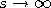, of the laws of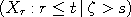.qmart1.ps.gzqmart1.pdf

On a parabolic Harnack inequality for Markov chains (1998)
abstract: For continuous time Markov chains on a countable state space, we derive a parabolic Harnack inequality using probabilistic methods. We derive some consequences of this inequality for the compactness of parabolic (\ie spacetime harmonic) functions of the process.parabolic.ps.gzparabolic.pdf

A quasi-ergodic theorem for evanescent processes (1997)
coauthored with Gareth Roberts
abstract: We prove a conditioned version of the ergodic theorem for Markov processes, which we call a quasi-ergodic theorem. We also prove a convergence result for conditioned processes as the conditioning event becomes rarer.ergo2_rev.ps.gzergo2_rev.pdf

Quasistationarity and Conditioned Markov Processes (1997)
abstract: Ph.D. thesis.phd-thesis.ps.gzphd-thesis.pdf

Quasistationary theorems for diffusions in a bounded open set (1996)
abstract: Letbe the minimal diffusion associated with a uniformly elliptic differential operator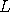in a bounded subdomain of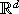, with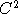boundary. Under the only assumption that the coefficients ofbe Hölder continuous, we prove all the standard quasistationary limit theorems (cf. Markov chain theory). Moreover, we show that the laws of, conditioned on explosion occuring after time, converge in total variation, as s tends to infinity, to the law of a positive recurrent diffusion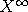, which is related toby the addition of the drift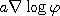, where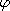is the ground state of. Previously, such results were shown only for symmetrically reversible diffusions.multidiffb.ps.gzmultidiffb.pdf

Approximations of quasistationary distributions for Markov chains (1996)
coauthored with Andrew Hart
abstract: We consider a simple and widely used method for evaluating quasistationary distributions of continuous time Markov chains. The infinite state space is replaced by a large, but finite approximation, which is used to evaluate a candidate distribution. We give some conditions under which the method works, and describe some important pitfalls.approxr.ps.gzapproxr.pdf

Honours project: A tutorial on stochastic calculus (1994)
abstract: Fourth year honours project report. An introduction to stochastic calculus with a detailed bibliography.hon-thesis.ps.gzhon-thesis.pdf

 sitemap software essays mathematics unix links